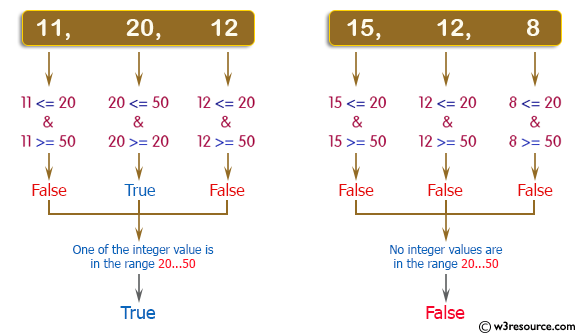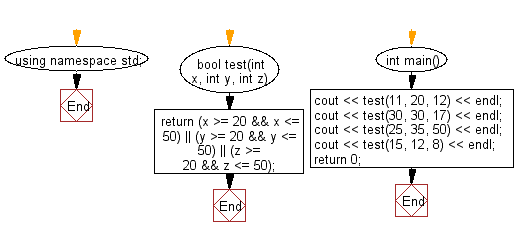﻿ C++ : Check if 3 values are in the range 20..50 inclusive# C++ Exercises: Check whether three given integer values are in the range 20..50 inclusive

## C++ Basic Algorithm: Exercise-15 with Solution

Write a C++ program to check whether three given integer values are in the range 20..50 inclusive. Return true if 1 or more of them are in the said range otherwise false.

Sample Solution:

C++ Code :

``````#include <iostream>
using namespace std;

bool test(int x, int y, int z)
{
return (x >= 20 && x <= 50) || (y >= 20 && y <= 50) || (z >= 20 && z <= 50);
}

int main()
{
cout << test(11, 20, 12) << endl;
cout << test(30, 30, 17) << endl;
cout << test(25, 35, 50) << endl;
cout << test(15, 12, 8) << endl;
return 0;
}
``````

Sample Output:

```1
1
1
0
```

Pictorial Presentation:Flowchart:C++ Code Editor:

Contribute your code and comments through Disqus.

What is the difficulty level of this exercise?

﻿